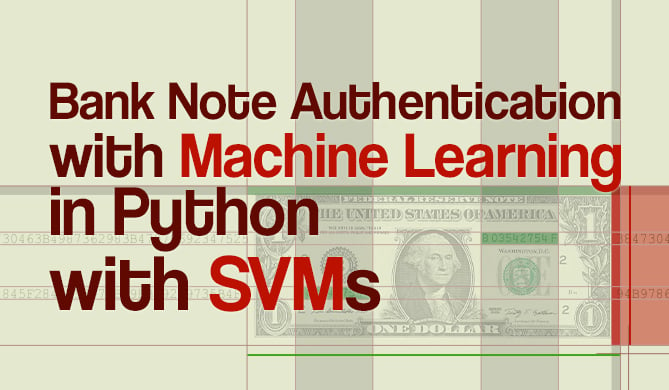End-to-End Gradient Boosting Regression Pipeline with Scikit-Learn

# End-to-End Gradient Boosting Regression Pipeline with Scikit-LearnRegression is a technique in statistics and machine learning, in which the value of an independent variable is predicted by its relationship with other variables.

Frameworks like Scikit-Learn and XGBoost make it easier than ever to perform regression with a wide variety of models - one of the recently well-adopted one being Gradient Boosting. While XGBoost has been gaining popularity and works well with Scikit-Learn - you can also use Scikit-Learn's own implementation of gradient boosting (which is around on par with XGBoost).

XGBoost works well with Scikit-Learn, has a similar API, and can in most cases be used just like a Scikit-Learn model - so it's natural to be able to build pipelines with both libraries. If you'd like to use it, read our "End-to-End XGBoost Regression Pipeline with Scikit-Learn"

With Scikit-Learn pipelines, you can create an end-to-end pipeline in as little as 4 lines of code: load a dataset, perform feature scaling, and then feed the data into a regression model:

from sklearn import datasets
from sklearn.model_selection import train_test_split
from sklearn.preprocessing import MinMaxScaler
from sklearn.pipeline import Pipeline

X, y = datasets.fetch_california_housing(return_X_y=True)
X_train, X_test, y_train, y_test = train_test_split(X, y)

pipeline = Pipeline([('scaler', MinMaxScaler()), ('regressor', GradientBoostingRegressor())])
pipeline.fit(X_train, y_train)

r2 = pipeline.score(X_test, y_test)
print(f"GBR: {r2}") # GBR: 0.783733539514218


Alternatively, you can separate the steps outside of the pipeline, which is a bit more verbose, yet more flexible:

X, y = datasets.fetch_california_housing(return_X_y=True)
X_train, X_test, y_train, y_test = train_test_split(X, y)

scaler = MinMaxScaler()
X_train_scaled = scaler.fit_transform(X_train)
X_test_scaled = scaler.transform(X_test)

r2 = gbr.score(X_test_scaled, y_test)
print(f"GBR: {r2}") # GBR: 0.7783900184162397

Last Updated: July 1st, 2022
David LandupAuthor

Entrepreneur, Software and Machine Learning Engineer, with a deep fascination towards the application of Computation and Deep Learning in Life Sciences (Bioinformatics, Drug Discovery, Genomics), Neuroscience (Computational Neuroscience), robotics and BCIs.

Great passion for accessible education and promotion of reason, science, humanism, and progress.Project

### Bank Note Fraud Detection with SVMs in Python with Scikit-Learn

# python# machine learning# scikit-learn# data science

Can you tell the difference between a real and a fraud bank note? Probably! Can you do it for 1000 bank notes? Probably! But it...Details